Next: 4 Surface Brightness Distribution Up: A Statistical Model of Previous: 2 Number Counts

# 3 Angular Size

Since galaxies are not sharp-edged objects, their angular size can be variously defined (see [Mihalas and Binney(1981)] and [Binney and Merrifield(1998)]). As far as studies of surface brightness distribution are concerned, however, the parameter most widely used to characterize the size of a galaxy is its effective radius. This can be roughly defined as the radius encircling half of the light emitted by the galaxy, but in practice its measurement is usually performed through a more complicated process.

Surface photometry of galaxies (see e.g. [Jedrzejewski(1987)] for E galaxies and [Kent(1985)] for D galaxies) is usually analysed by fitting ellipses to the isophotes and by plotting their surface brightness versus their radius, which is defined as the geometric mean of the ellipse's semi-axes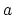and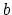, i.e.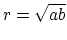. The resulting plot is then called the surface brightness radial profile of the galaxy. In this context, the effective radius of the galaxy is defined as the radius of the isophote encircling half of the light emitted by the galaxy, also called the effective isophote. The effective radius and the effective surface brightness, the latter being the surface brightness of the effective isophote, are usually indicated with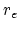and, respectively.

Until the launch of HST, accurate measurements of the small angular sizes of faint galaxies were made virtually impossible by the phenomenon of seeing. The Medium Deep Survey ([Ratnatunga et al.(1999)]), the first survey project to be carried out with HST superb instrumentation, has recently brought to an end this long-standing lack of meaningful data, while [Im et al.(1995)] have demonstrated the potential of angular size measurements to discriminate between currently competing cosmological models.

[Casertano et al.(1995)] have obtained effective radii for about 10,000 galaxies from Wide Field and Planetary Camera (WF/PC) parallel observations of random fields in the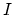band. As shown in their Figure 6, the observed angular size distribution as function ofmagnitude shows a large scatter about the median value, mainly due to the intrinsic scatter in linear size and redshift distribution. The same figure also shows that the observed relation between the median effective radius andmagnitude is well-fit by the theoretically predicted relation for galaxies of constant central surface brightness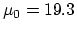mag/arcsec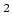and absolute magnitude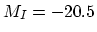in the context of a mild luminosity evolution scenario. This latter relation asymptotically approaches the linear relation in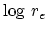vs.that is measured in local samples of bright spiral galaxies following Freeman's law, and was therefore taken as a description of the relation between the galaxy effective radius and magnitude in our model. Least-square polynomial fit showed that its accurate description required a fourth-degree polynomial, which is represented in Figure 3, together with the Euclidean extrapolation to faint magnitudes of the local linear relation.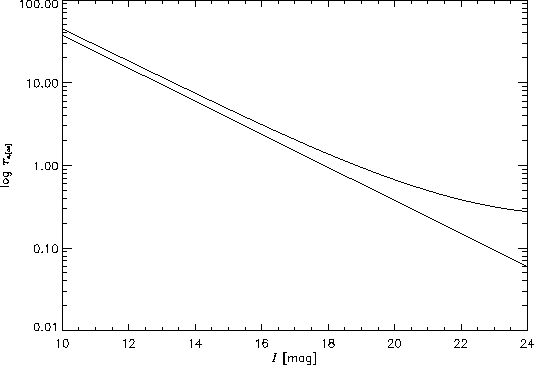The relation that in the following will be used to expressas function ofis therefore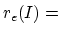dex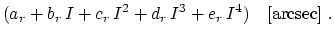(3)

The values of the five parameters contained in Equation 3 are given in Table 3.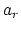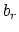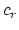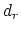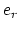3.57702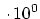-2.12805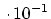5.34616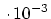-4.62001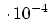1.28947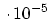Note that it is assumed not only thatdepends ononly, but also that the same relation holds for all galaxies, irrespective of their morphological types. From a statistical point of view, however, and as far as Equation 3 holds on average, the net effect on the total number of detected galaxies should be negligible. Effective radii calculated from Equation 3, are given in Table 4. A rough estimation of the typical surface brightness of the central regions of galaxies can be given by the average surface brightness inside the effective radius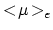. Under our assumptions, and from the definition of effective radius, this quantity is equal for Es and Ds and can be written as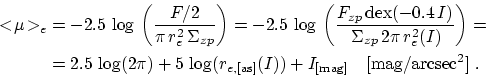(4)

Values ofare given in Table 5. To characterize the total fraction of the sky occupied by galaxies brighter than a given magnitude, one can define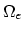as the total solid angle that lies within the effective radius of all galaxies brighter than a given magnitude. Since superposition of different galaxies on the same sky regions is negligible, at least in the magnitude range we are considering,can simply be written as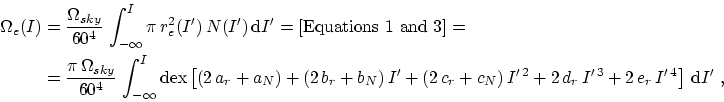(5)

where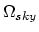is the solid angle spanned by the whole sky and the factor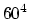was introduced to take into account the fact that the coefficients giving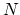andas function ofin Equations 1 and 3 are expressed in different angular units. Values of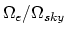, calculated through Romberg integration of Equation 5, are given in Table 4.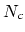mag deg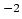mag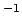degarcsec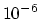sky 10 0.008183 0.005151 44.72 5.350 11 0.03777 0.02458 28.64 10.93 12 0.1654 0.1114 18.29 21.15 13 0.6867 0.4791 11.68 38.88 14 2.705 1.958 7.468 68.08 15 10.10 7.596 4.801 114.0 16 35.79 27.99 3.115 183.6 17 120.3 98.00 2.049 285.9 18 383.3 325.9 1.374 433.3 19 1159 1030 0.9446 644.0 20 3322 3093 0.6707 946.5 21 9032 8826 0.4954 1389 22 23290 23940 0.3838 2060 23 56970 61760 0.3147 3132 24 132200 151500 0.2758 4969Next: 4 Surface Brightness Distribution Up: A Statistical Model of Previous: 2 Number Counts
Mattia Vaccari 2002-01-31